Research ArticleJournal of Agricultural, Life and Environmental Sciences. December 2019. 143-150
https://doi.org/10.22698/jales.20190017

# MAIN

• Introduction

• Materials and Methods

•   Image acquisition

•   Extraction of meeting ground between each image

•   Statistical Analysis

• Results and Discussion

## Introduction

In order to evaluate crop status in the field, a large amount and various types of information are required. Traditional crop evaluation method in the field is manually taking samples of crops, resulting in destructive, time-consuming, and laborious. However, remote sensing technique can acquire crop data with non-destructive and non-laborious methods rapidly (Lillesand et al., 2015). With the development of high performing sensors and platforms, remote sensing become a promising technique. Among remote sensing platforms, aerial platforms including satellite, manned aircraft and unmanned aerial vehicle (UAV) can provide a high-throughput data acquisition in field detection. Recently, remote sensing using UAV platform has been studied a lot due to the advantages of UAV platform which provide high spatial and temporal resolution inexpensively compared to satellite and manned aircraft (Muchiri and Kimathi, 2016; Shi et al., 2016).

However, the complexity of Earth’s components such as the land and atmosphere and condition of illumination can affect data acquisition with various errors such as atmospheric effect, geometric error and radiometric error (Shahtahmassebi et al., 2013). Especially, shadows are major sources causing radiometric problem in the field with false color tone of vegetation. Moreover, shadows reduce the success of image processing for extraction of crop information, interrupting feature extraction, image matching, and change detection (Junli and Shugen, 2002; Singh et al., 2012). Thus, the adverse effect of shadows is required to be analyzed for the generation of high-quality image data. However, there is a little research in remote sensing using the UAV platform despite of active study in using satellite (Yamazaki et al., 2009; Liu and Yamazaki, 2012; Singh et al., 2012; Milas et al., 2017). In the current paper, the effects of shadow in RGB images using the UAV platform are reported to improve image data remotely sensed by the UAV platform so that researchers can be aware of this issue.

## Materials and Methods

Image acquisition

The shadowing image and no-shadowing image by cloud were acquired using an RGB camera mounted on the UAV platform (Mavic 2 Zoom, DJI, China) on 26 July 2019 (Table 1). The images were captured on the waypoint path automatically set by Pix4Dcapture (Pix4D SA, Lausanne, Switzerland), UAV flight planning application. The shadowing image was capture immediately after the no-shadowing image (Fig. 1). The test field is located at Jeju National University (33°72'N, 126°33'E, altitude 257 m), 102, Jejudaehak-ro, Jeju-si, Jeju-do, Republic of Korea.

Table 1. Specification of the RGB camera mounted on the UAV platform (Mavic 2 Zoom) used in the study

 Item Specification Resolution 4000 × 3000 Image sensor 1/2.3" CMOS Focal length 24‒48 mm Field of view 72.3° (horizontal), 57.5° (vertical) F-stop (aperture) 2.8‒3.8 Shutter speed 8‒1/8000 s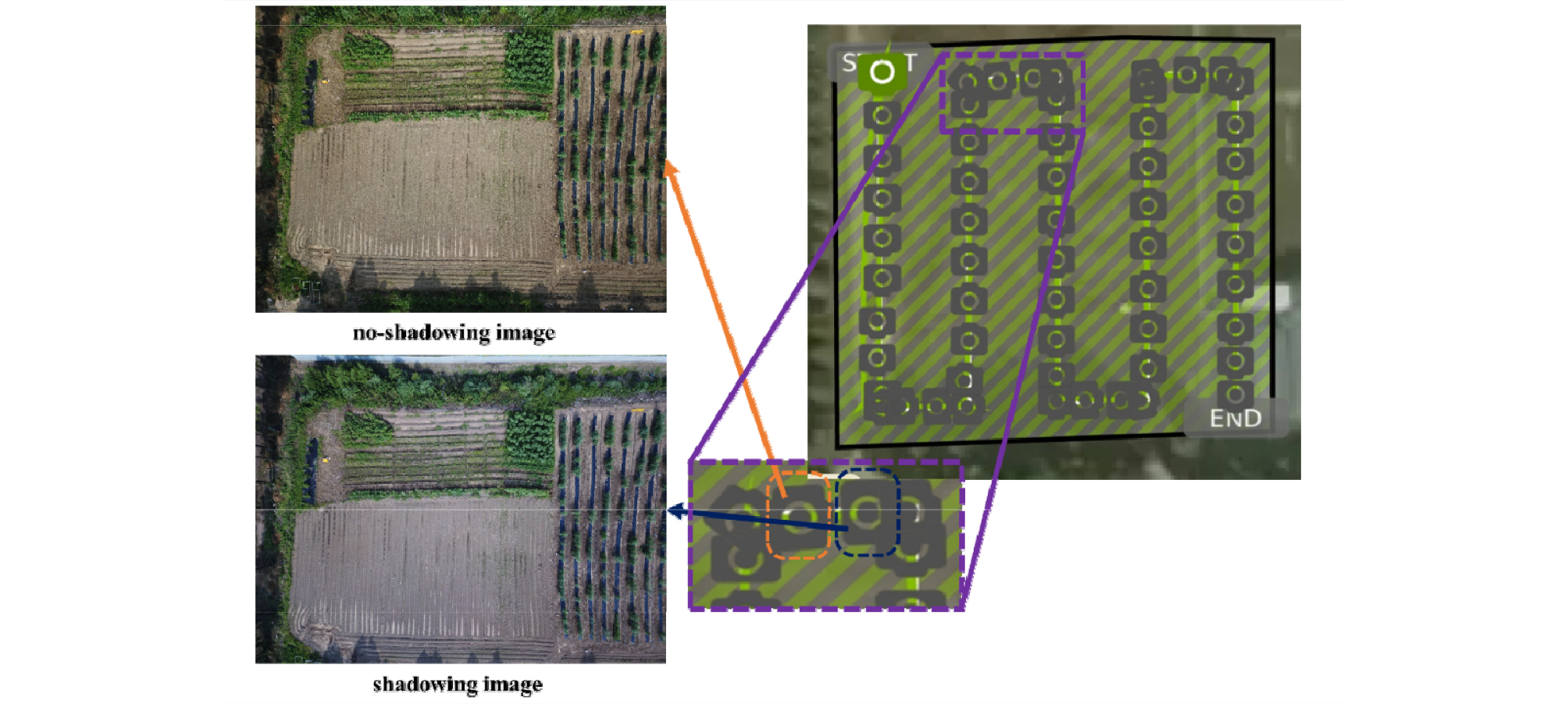##### Fig. 1.

The waypoint path set by ‘Pix4Dcapture’ software and the acquired no-shadowing image and shadowing image.

The conditions of illumination and atmosphere are ever-changing, resulting in the radiometric errors of the image captured by an RGB camera. Thus, the digital number (DN) of the RGB camera should convert to the standard reflectance. In this study, the empirical line method was used for radiometric calibration (Smith and Milton, 1999). Calibration targets (1.2 by 1.2 m Group 8 Technology Type 822 ground calibration panels), which provide the standard reflectance value with four scales (3%, 12%, 36%, and 56%) were placed in the test field (Fig. 2A). The DNs of each red, green, and blue band were extracted from the image, including the calibration target by ArcGis 10.6 (ESRI, Redlands, USA), geographic information system software. The extracted DNs were fitted to the reflectance value, generating equation (1). On 26 July 2019, the generated exponential regression model in each band was shown in (Fig. 2C). Finally, the equation was applied to the DNs in both shadowing image and no-shadowing image converting to the standard reflectance value.

 $$r_k=A_ke^{B_kDN}$$ (1)
where, represents the reflectance values of the acquired images, DN represents the digital number for each band, and and are the coefficients of the exponential regression model.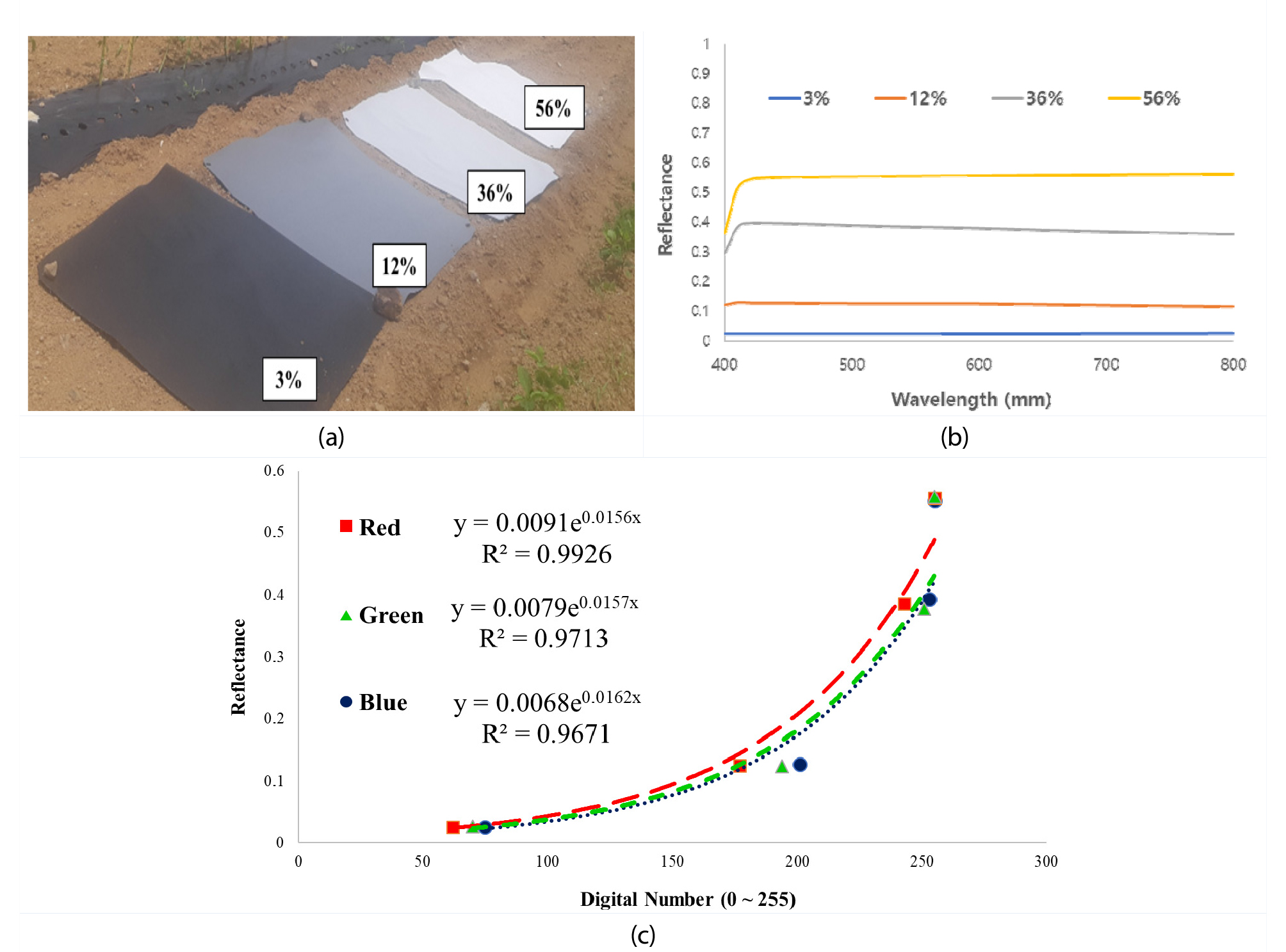##### Fig. 2.

(a) Calibration targets in the field; (b) Standard reflectance spectrum of the calibration targets; (c) Exponential regression model of the red, green, and blue band on 26 July 2019.

Extraction of meeting ground between each image

Shadowing image and no-shadowing were captured at different sites on the straight travel of the UAV platform (Fig. 3). Thus, the meeting ground between each image was extracted by the calculation of the differences of the x-axis and y-axis distance. In each image, white and black panel providing the position indicatable by 1 pixel on its center was appeared in common (Fig. 3). Based on the position of white and black panel’s center, the differences of the x-axis and y-axis distance were calculated to 10 pixels and 274 pixels respectively. That means the shadowing image was captured at the position in the x-axis direction, -10 pixels and in the y-axis direction, 274 pixels from the no-shadowing image. As a result, the meeting ground was extracted as 3990 pixels in the x-axis and 2726 pixels in the y-axis (Fig. 4) within each image.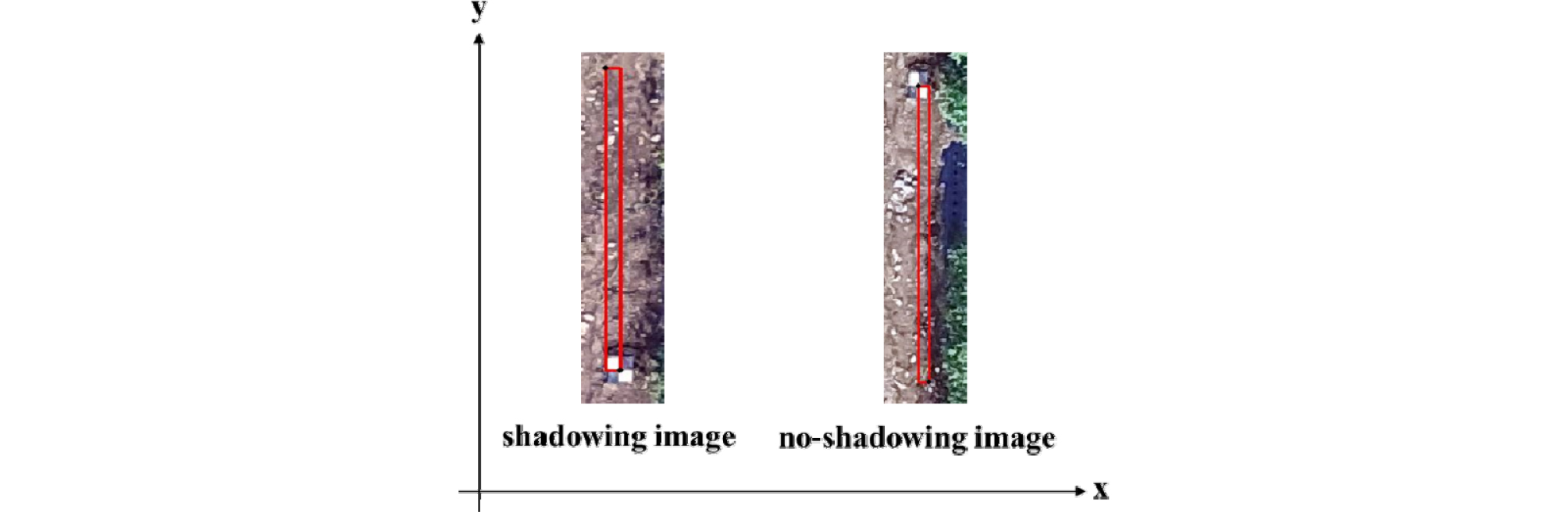##### Fig. 3.

Calculation of pixel difference (x-axis and y-axis) between no- shadow image and shadow image using white and black panel.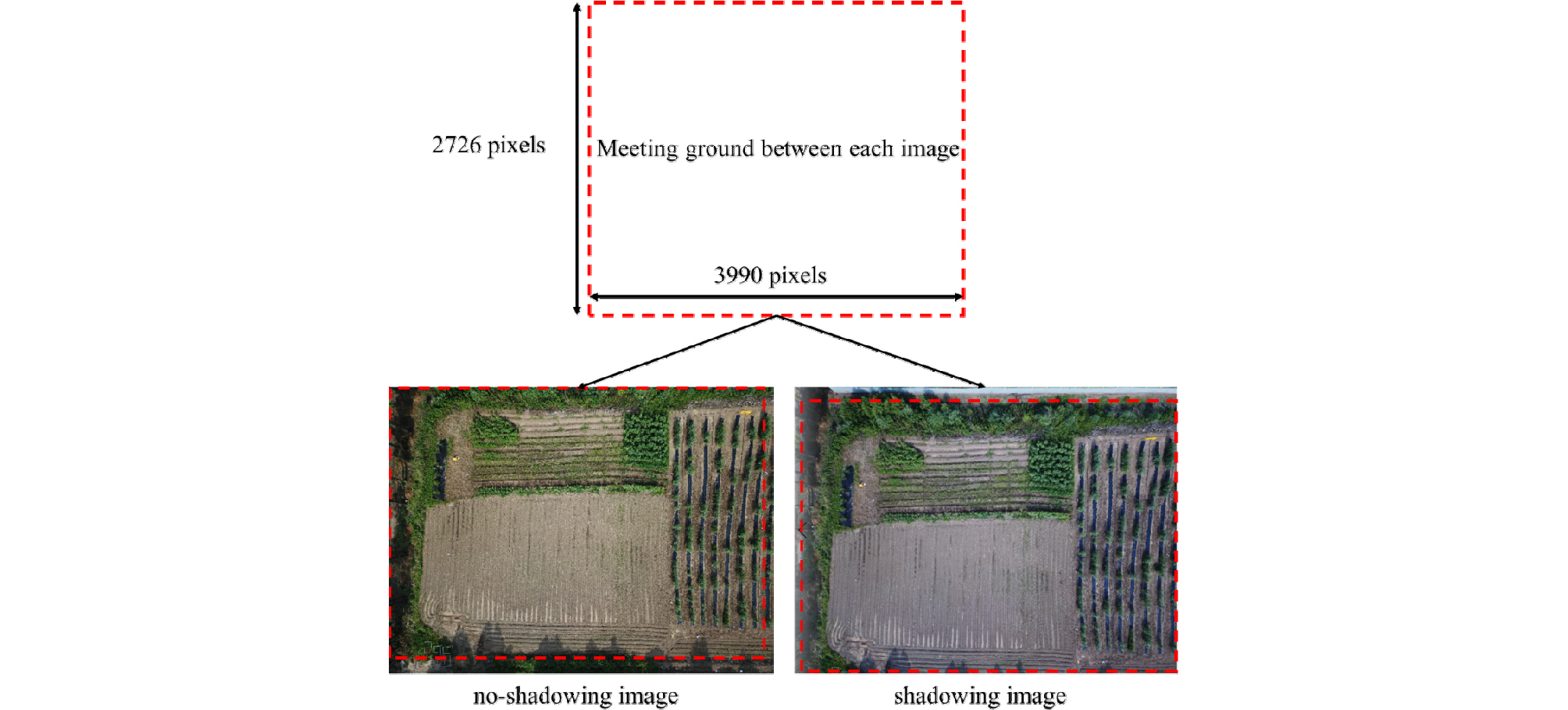##### Fig. 4.

Extraction of the meeting ground between no-shadow image and shadow image and comparison of the pixel value of each band.

Statistical Analysis

In the current study, the shadow image was compared to the on-shadowing image (Fig. 5). Statistical analysis consisted of fitting Bayesian linear regression to verify the association between the presence or absence of the shadows and the color channels (red, green, and blue). The statistical model follows:

 $$y_i=\beta_0+\beta_1x_i+\epsilon_i$$ (2)

where, ${\beta }_{0}$ is the intercept, ${\beta }_{1}$ is the slope of straight line, $ϵ~N\left(0,{\sigma }^{2}\right)$.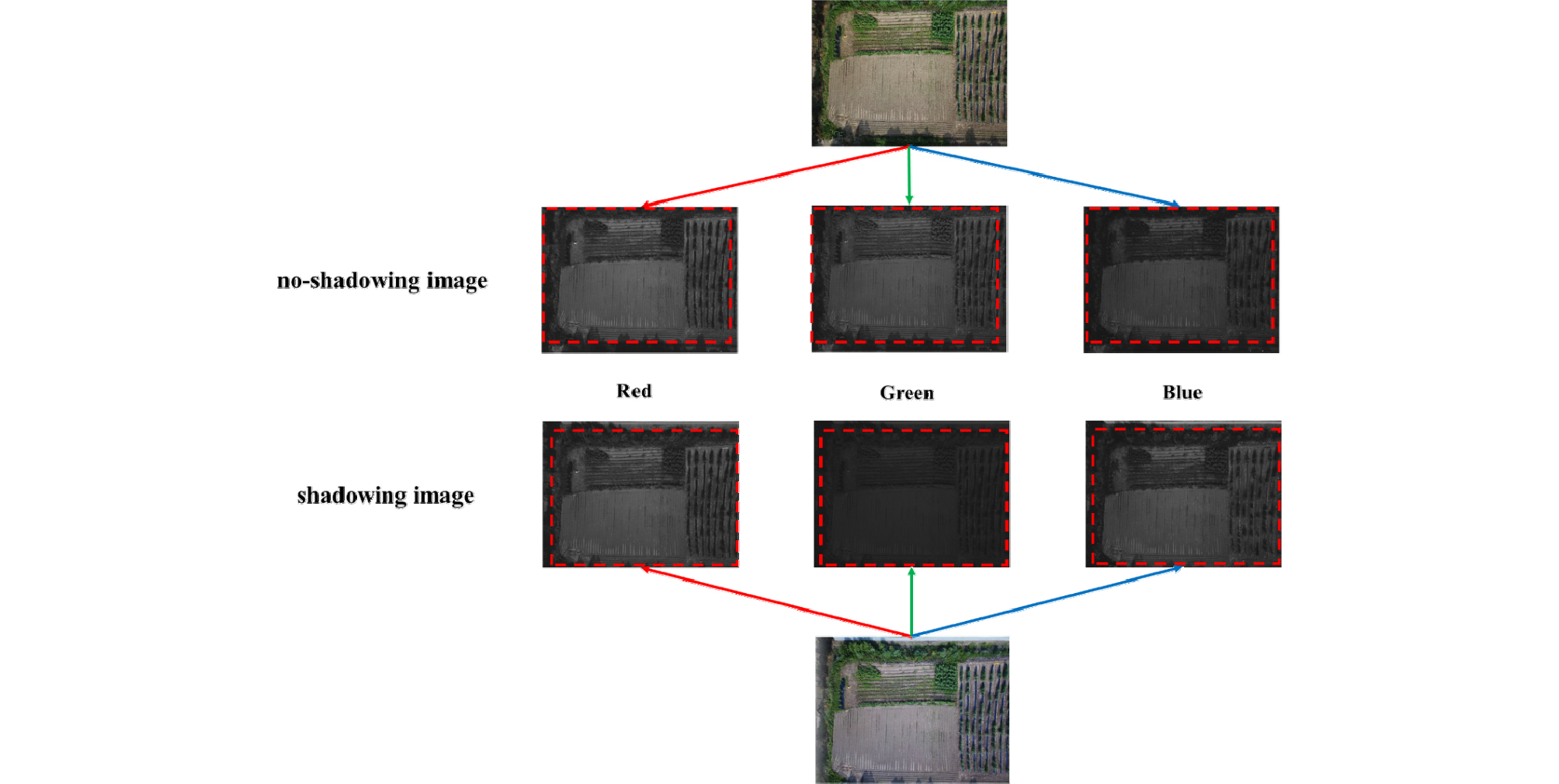##### Fig. 5.

Analysis and comparison between each red, green, and blue band of no-shadowing image and shadowing image processed with radiometric calibration.

Vague priors are assumed for regression coefficients and error variance.

 $$\beta_j\sim N(0,\tau_j^2),11j=1,2$$ (3)

where, ${\tau }_{j}^{2}=1000$ and

 $$\frac1{\sigma^2}\sim\Gamma(\frac{c_0}2,\frac{d_0}2)$$ (4)

where, ${c}_{0}={d}_{0}=0.002$.

Significance of the shadows effects was tested using the Bayes factor. In an overview, the Bayes factor measure which model is more plausible given the data (Gelman et al., 2014).

 $$B_{12}=\frac{p(y\left|M_1)\right.}{p(y\left|M_2)\right.}\;\Rightarrow\log B_{12}=\log pp(y\left|M_1)-\log pp(y\left|M_2)\right.\right.$$ (5)

where, ${B}_{12}$ is the Bayes factor, are models 1 and 2, respectively. All computations were done using R statistical software (R Core Team, 2018) and MCMCpack R package (Martin et al., 2011).

## Results and Discussion

Box plots of the distribution of RGB values suggest that shadow effects are significant for colors blue and green, but for red color the difference between the two groups is not quite high (Fig. 6). However, Bayes factor results reveal that models with shadow effects included in the linear predictor is more likelihood, i.e, the shadows effect is significant for all channel colors (Table 2). Interestingly, the shadow effect is less for red color, unlike green and blue. This could be because the compared images were soil, which contains red color more than others. It might have different results if vegetative images were compared. It could be worth to compare only between vegetative areas to determine shadow effects.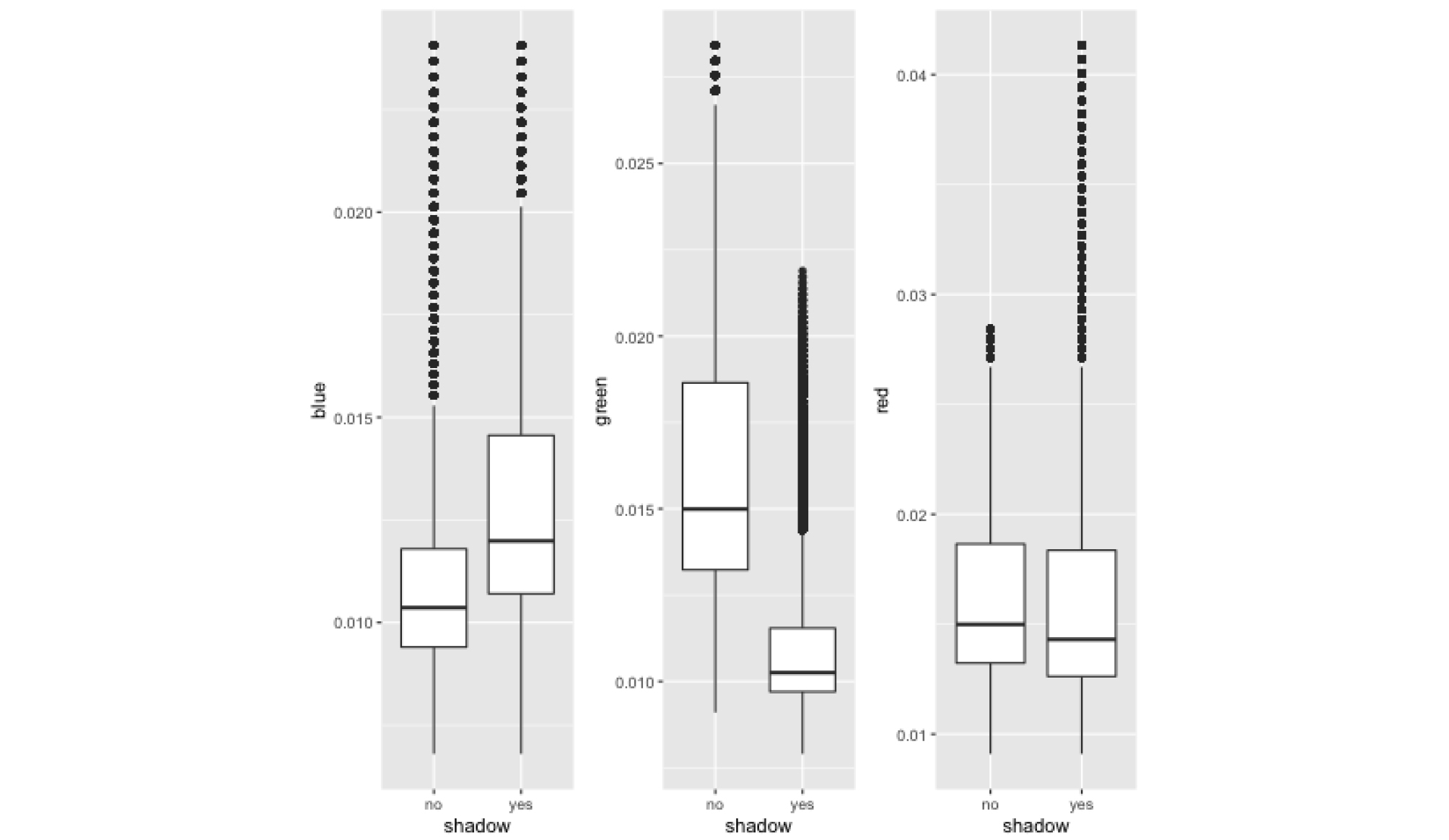##### Fig. 6.

Box plots of the distribution of red, green, and blue band values with presence and absence of the shadow.

Table 2. Posterior means of the shadow effect and standard deviation from the posterior distribution of the shadow effects, and the natural logarithm Bayes Factor

 Models Shadow effect Standard deviation log Bayes Factor Blue 0.0023 <0.0001 79671 Green -0.0056 <0.0001 319730 Red 0.0001 <0.0001 55.8

The current study examined the shadow effects in the image analysis captured from UAV. Considering the current research trend using UAV, this issue is very important to know to perform experiments properly. Thus, we report this result with excitement to help researchers who do field experiments with UAV derived image analysis.

## Acknowledgements

This research was supported by Basic Science Research Program through the National Research Foundation of Korea (NRF) funded by the Ministry of Education (NRF-2019R1F1A1061916). Also, we thank Sustainable Agriculture Research Institute (SARI) in Jeju National University for providing the experimental facilities.

## References

1

Gelman, A., Carlin, J. B., Stern, H. S., Rubin, D. B. (2014) Bayesian data analysis: Chapman & Hall/CRC Boca Raton, FL, USA.

2

Junli, W., Shugen, W. (2002) A method of image shadow detection based RGB colour space. Inf Technol 26:7-9.

3

Lillesand, T., Kiefer, R. W. Chipman, J. (2015) Remote sensing and image interpretation, John Wiley and Sons, New York, NY, USA.

4

Liu, W., Yamazaki, F. (2012) Object-based shadow extraction and correction of high-resolution optical satellite images. IEEE J Sel Topics Appl Earth Observ Remote Sens 5:1296-1302.

10.1109/JSTARS.2012.2189558
5

Martin, A. D, Quinn, K. M., Park, J. H. (2011) MCMCpack: Markov Chain Monte Carlo in R. J Stat Softw 42:1-21.

10.18637/jss.v042.i09
6

Milas, A. S., Arend, K., Mayer, C., Simonson, M. A., Mackey, S. (2017) Different colors of shadows: classification of UAV images. Int J Remote Sens 38:3084-3100.

10.1080/01431161.2016.1274449
7

Muchiri, N., Kimathi, S. (2016) A review of applications and potential applications of UAV. Proceedings of Sustainable Research and Innovation Conference 280-283.

8

R Core Team (2018) R: A language and environment for statistical computing [Internet]. Vienna, Austria: R Foundation for Statistical Computing.

9

Shahtahmassebi, A., Yang, N., Wang, K., Moore, N., Shen, Z. (2013) Review of shadow detection and de-shadowing methods in remote sensing. Chin Geogr Sci 23:403-420.

10.1007/s11769-013-0613-x
10

Shi, Y., Thomasson, J. A., Murray, S. C., Pugh, N. A., Rooney, W. L., Shafian, S., Rajan, N., Rouze, G., Morgan, C. L., Neely, H. L., Rana, A., Bagavathiannan, M. V., Henrickson, J., Bowden, E., Valasek, J., Olsenholler, J., Bishop, M. P., Sheridan, R., Putman, E. B., Popescu, S., Burks, T., Cope, D., Ibrahim, A., McCutchen, B. F., Baltensperger, D. D., Avant Jr, R. V., Vidrine, M., Yang, C. (2016) Unmanned aerial vehicles for high-throughput phenotyping and agronomic research. PLoS One 11: e0159781.

10.1371/journal.pone.015978127472222PMC4966954
11

Smith, G. M., Milton, E. J. (1999) The use of the empirical line method to calibrate remotely sensed data to reflectance. Int J Remote Sens 20:2653-2662.

10.1080/014311699211994